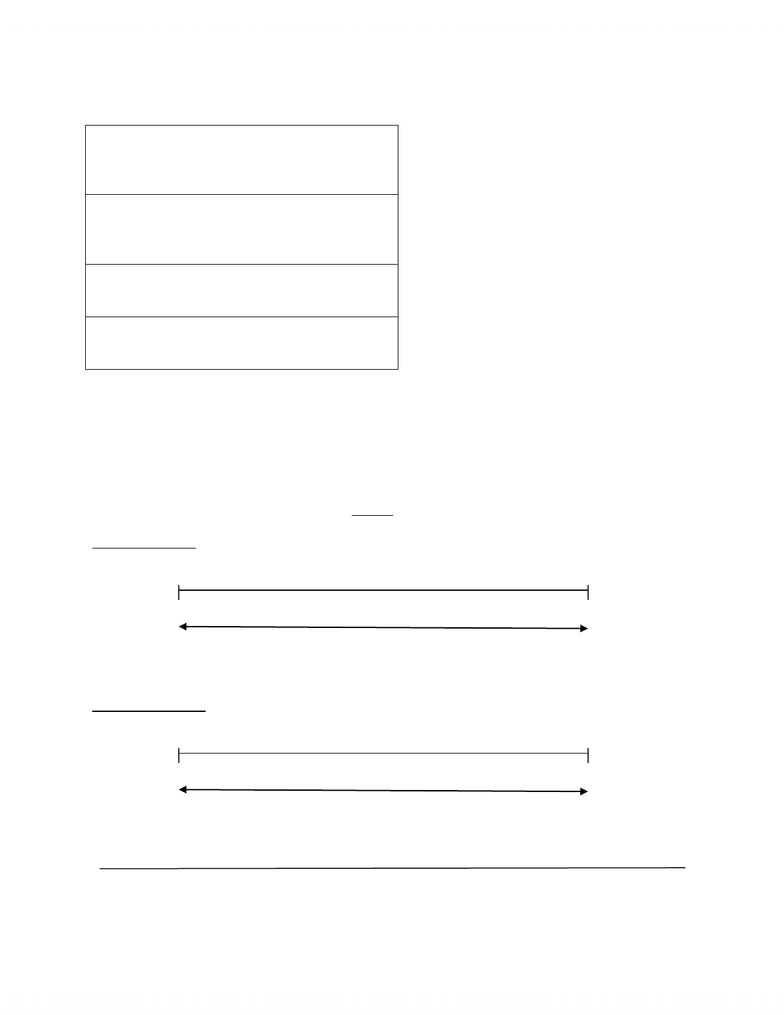# CHEM 1114 Lecture Notes - Sodium Hydroxide, Acid Strength, Decimal Mark

122 views7 pages23 Mar 2013
School
Department
Course
ProfessorACID, BASES & SALTS
Acid: any compound which produces hydrogen ions (H+) in solution
Base: any compound which produces hydroxide ions (OH-) in solution
Salt: any compound which is the neutralization of an acid and base
When a chemical donates Hydrogen, it is an acid. When a chemical receives Hydrogen, it is a base.
Ex. CO 3
2- + H2O  HCO3
- + OH-
BASE ACID BASE ACID
H2O donates H+, so it is an ACID
CO3
2 accepts H+, so it is a BASE
A reaction can occur between 2 water molecules in pure water. This is called the self- ionization of water
Ex. 59KJ +H2O+ H2O  H3O+ + OH-
The Kw = [H3O][OH-] is the equilibrium constant for water at 25o C , Kw =1.0 x 10-14
At 25oC Kw = [H3O][OH-] = 1.0 x 10-14
X2 = 1.0 x 10-14
X= [H3O] = [OH-] = 1.0 x 10-7 M
Temperature Effect on the Kw
-an increase in temperature favours the PRODUCTS and favours the formation of ions
- water remains neutral because [H3O] = [OH-]
Solutions are classified as acidic/ basic/neutral according to the relative concentrations of H3O+
And OH- present
IF...
[H3O+] > [OH-] , solution is ACIDIC
[H3O+] < [OH-] , solution is BASIC
[H3O+] = [OH-] , solution is NEUTRAL
Unlock document

This preview shows pages 1-2 of the document.
Unlock all 7 pages and 3 million more documents.NOTE: [H+] = [OH-]
pH = -log [H+]
[H+] = antilog (-pH) = 10-pH
pOH= -log [OH-]
[OH-]= antilog (-pOH)
pKw = pH +pOH where pKw = -log Kw
At 25oC , ph+ pOH = 14.00
Ex. What is the pH of a solution that contains [H+] = 3.2 x 10-9 M?
pH = - log [H+] = -log (3.2 x 10-9)
pH = 8.49 (only 2 sig figs. )!
NOTE : In a pH/pOH/pKw value, the numbers before the decimal point does not count as sig figs
pH scale (at 25oC) *when H3O increases, pH decreases
[ H3O+] 1x10-1 1x10-4 1x10-7 1x10-10 1x10-14
pH 1 4 7 10 14
acidic neutral basic
NOTE: It is possible to have pH values that are negative and to have ones that exceed 14
pOH scale (at 25oC ) *when H3O decreases, pOH increases
[OH-] 1x10-1 1x10-4 1x10-7 1x10-10 1x10-14
pOH 1 4 7 10 14
basic neutral acidic
Unlock document

This preview shows pages 1-2 of the document.
Unlock all 7 pages and 3 million more documents.

## Document Summary

Acid: any compound which produces hydrogen ions (h+) in solution. Base: any compound which produces hydroxide ions (oh-) in solution. Salt: any compound which is the neutralization of an acid and base. When a chemical donates hydrogen, it is an acid. When a chemical receives hydrogen, it is a base. H2o donates h+, so it is an acid. 2 accepts h+, so it is a base. A reaction can occur between 2 water molecules in pure water. This is called the self- ionization of water. The kw = [h3o][oh-] is the equilibrium constant for water at 25o c , kw =1. 0 x 10-14. X= [h3o] = [oh-] = 1. 0 x 10-7 m. An increase in temperature favours the products and favours the formation of ions. Water remains neutral because [h3o] = [oh-] Solutions are classified as acidic/ basic/neutral according to the relative concentrations of h3o+ Note: [h+] = [oh-] ph = -log [h+]

# Get access

\$10 USD/m
Billed \$120 USD annually
Homework Help
Class Notes
Textbook Notes